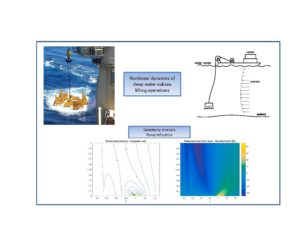# Nonlinear dynamics of deep water subsea lifting operations considering KC-dependent hydrodynamic coefficients.￼

The dynamics of deep water subsea lifting operations considering hydrodynamic coefficients that depend on the Keulegan-Carpenter () number are analysed in this study. Firstly, experimental data from the literature is presented for a typical subsea manifold, relating the added mass and drag coefficients to the amplitude of oscillation, represented by the number. Then, the nonlinear non-dimensional equation of motion, that considers the variable hydrodynamic coefficients, is presented. The solution of this equation is obtained via the harmonic balance method and by iterative time domain integration, and the results are compared to those of a conventional model with constant hydrodynamic coefficients. The results obtained via the harmonic balance method are considered almost as accurate as from the time domain integration, but require significantly less computational effort. Also, it is shown that the amplitude-dependent model predicts variations in the natural frequency and damping of the system as a function of the amplitude of the response of the payload. This results in significant differences in the maximum cable tension and the payload depth at which it occurs, compared with the constant hydrodynamic coefficient model. Hence this shows the importance of considering variable hydrodynamic coefficients when analysing subsea lifting systems.Tommasini R. B., Hill T.L., Macdonald J.H.G., Pavanello R., Carvalho L.O. , Nonlinear dynamics of deep water subsea lifting operations considering KC-dependent hydrodynamic coefficients. Ocean Engineering, vol. 233, pp. 109172, 2021.